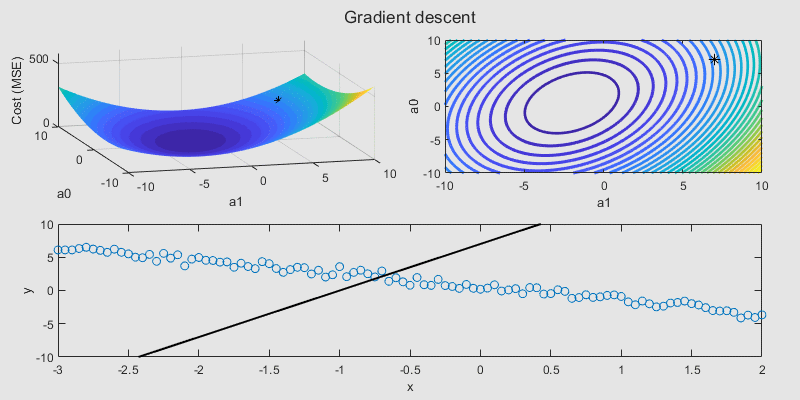## Regression Basics

versión 2.0.2 (306 KB) por
Interactive courseware module that addresses the fundamentals of regression analysis taught in STEM courses.

371 descargas

Actualizada 26 Aug 2022

De GitHub

# Regression Basics

Curriculum Module
Created with R2020a. Compatible with R2020a and later releases.

## Description

This package contains a live script and supporting files to illustrate some basics of regression analysis. The materials are designed to be flexible and can be easily modified to accommodate a variety of teaching and learning methods. We include a brief background, interactive illustrations, tasks, reflection questions, a real-world application example, and a guided exercise for the different concepts explored.

The instructions inside the live scripts will guide you through the tasks and activities one section at a time. To run this interactive script in a controls-only mode, use the Hide code button on the View tab of the MATLAB toolstrip.

Learning Goals

• Explain the difference between linear, multiple linear, and nonlinear regression.
• Use ordinary least squares to analytically solve for linear regression parameters.
• Assess and improve the performance of a regression model using a goodness-of-fit measure.
• Apply gradient descent to iteratively minimize a cost function and estimate model parameters.
• Explain the effect of increasing and decreasing the learning rate and number of steps for the gradient descent algorithm.
• Use a linear regression model to perform short-term forecasting.## Suggested Prework

MATLAB Onramp – a free two-hour introductory tutorial to learn the essentials of MATLAB®.

## Details

`regressionBasics.mlx`An interactive lesson that introduces the fundamentals of regression analysis. Students apply a basic linear regression to model real-world electricity load data.

`electricityLoadData.mlx`A supplementary script to download the external electricity load data from New York ISO for use in the practice problem.

`regressSolnIm/`
This folder includes supplementary image files containing solutions for tasks in `regressionBasics.mlx`. The main script provides controls to hide or expose the solutions when needed. Ensure that this folder is in the same location as `regressionBasics.mlx`

`linearData.mat`, `linearData2.mat`, `multivariateData.mat`, `nonlinearData.mat`
Data files containing some sample data for the different types of regression problems.

## Products

MATLAB®, Statistics and Machine Learning Toolbox™

The license for this module is available in the LICENSE.TXT file in this GitHub repository.

## Support

Have any questions or feedback? Contact the MathWorks online teaching team.

Copyright 2021 The MathWorks, Inc.

### Citar como

Emma Smith Zbarsky (2022). Regression Basics (https://github.com/MathWorks-Teaching-Resources/Regression-Basics/releases/tag/v2.0.2), GitHub. Recuperado .

##### Compatibilidad con la versión de MATLAB
Se creó con R2020a
Compatible con la versión R2020a y siguientes
##### Compatibilidad con las plataformas
Windows macOS Linux

### Community Treasure Hunt

Find the treasures in MATLAB Central and discover how the community can help you!

Start Hunting!
Para consultar o informar de algún problema sobre este complemento de GitHub, visite el repositorio de GitHub.
Para consultar o informar de algún problema sobre este complemento de GitHub, visite el repositorio de GitHub.